# Best response

In game theory, the best response is the strategy (or strategies) which produces the most favorable outcome for a player, taking other players' strategies as given (Fudenberg & Tirole 1991, p. 29; Gibbons 1992, pp. 3349). The concept of a best response is central to John Nash's best-known contribution, the Nash equilibrium, the point at which each player in a game has selected the best response (or one of the best responses) to the other players' strategies (Nash 1950).

## Best response correspondence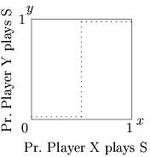Figure 1. Reaction correspondence for player Y in the Stag Hunt game.

Reaction correspondences, also known as best response correspondences, are used in the proof of the existence of mixed strategy Nash equilibria (Fudenberg & Tirole 1991, Section 1.3.B; Osborne & Rubinstein 1994, Section 2.2). Reaction correspondences are not "reaction functions" since functions must only have one value per argument, and many reaction correspondences will be undefined, i.e. a vertical line, for some opponent strategy choice. One constructs a correspondence, for each player from the set of opponent strategy profiles into the set of the player's strategies. So, for any given set of opponent's strategies,represents player i 's best responses to.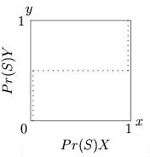Figure 2. Reaction correspondence for player X in the Stag Hunt game.

Response correspondences for all 2x2 normal form games can be drawn with a line for each player in a unit square strategy space. Figures 1 to 3 graphs the best response correspondences for the stag hunt game. The dotted line in Figure 1 shows the optimal probability that player Y plays 'Stag' (in the y-axis), as a function of the probability that player X plays Stag (shown in the x-axis). In Figure 2 the dotted line shows the optimal probability that player X plays 'Stag' (shown in the x-axis), as a function of the probability that player Y plays Stag (shown in the y-axis). Note that Figure 2 plots the independent and response variables in the opposite axes to those normally used, so that it may be superimposed onto the previous graph, to show the Nash equilibria at the points where the two player's best responses agree in Figure 3.

There are three distinctive reaction correspondence shapes, one for each of the three types of symmetric 2x2 games: coordination games, discoordination games and games with dominated strategies (the trivial fourth case in which payoffs are always equal for both moves is not really a game theoretical problem). Any payoff symmetric 2x2 game will take one of these three forms.

### Coordination games

Games in which players score highest when both players choose the same strategy, such as the stag hunt and battle of the sexes are called coordination games. These games have reaction correspondences of the same shape as Figure 3, where there is one Nash equilibrium in the bottom left corner, another in the top right, and a mixing Nash somewhere along the diagonal between the other two.

### Anti-coordination games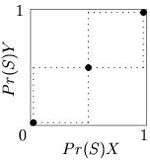Figure 3. Reaction correspondence for both players in the Stag Hunt game. Nash equilibria shown with points, where the two player's correspondences agree, i.e. cross

Games such as the game of chicken and hawk-dove game in which players score highest when they choose opposite strategies, i.e., discoordinate, are called anti-coordination games. They have reaction correspondences (Figure 4) that cross in the opposite direction to coordination games, with three Nash equilibria, one in each of the top left and bottom right corners, where one player chooses one strategy, the other player chooses the opposite strategy. The third Nash equilibrium is a mixed strategy which lies along the diagonal from the bottom left to top right corners. If the players do not know which one of them is which, then the mixed Nash is an evolutionarily stable strategy (ESS), as play is confined to the bottom left to top right diagonal line. Otherwise an uncorrelated asymmetry is said to exist, and the corner Nash equilibria are ESSes.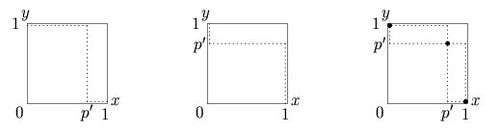Figure 4. Reaction correspondence for both players in the hawk-dove game. Nash equilibria shown with points, where the two player's correspondences agree, i.e. cross

### Games with dominated strategies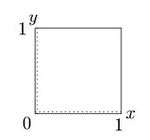Figure 5. Reaction correspondence for a game with a dominated strategy.

Games with dominated strategies have reaction correspondences which only cross at one point, which will be in either the bottom left, or top right corner in payoff symmetric 2x2 games. For instance, in the single-play prisoner's dilemma, the "Cooperate" move is not optimal for any probability of opponent Cooperation. Figure 5 shows the reaction correspondence for such a game, where the dimensions are "Probability play Cooperate", the Nash equilibrium is in the lower left corner where neither player plays Cooperate. If the dimensions were defined as "Probability play Defect", then both players best response curves would be 1 for all opponent strategy probabilities and the reaction correspondences would cross (and form a Nash equilibrium) at the top right corner.

### Other (payoff asymmetric) games

A wider range of reaction correspondences shapes is possible in 2x2 games with payoff asymmetries. For each player there are five possible best response shapes, shown in Figure 6. From left to right these are: dominated strategy (always play 2), dominated strategy (always play 1), rising (play strategy 2 if probability that the other player plays 2 is above threshold), falling (play strategy 1 if probability that the other player plays 2 is above threshold), and indifferent (both strategies play equally well under all conditions).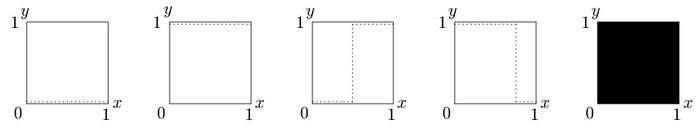Figure 6 - The five possible reaction correspondences for a player in a 2x2 game., The axes are assumed to show the probability that the player plays their strategy 1. From left to right: A) Always play 2, strategy 1 is dominated, B) Always play 1, strategy 2 is dominated, C) Strategy 1 best when opponent plays his strategy 1 and 2 best when opponent plays his 2, D) Strategy 1 best when opponent plays his strategy 2 and 2 best when opponent plays his 1, E) Both strategies play equally well no matter what the opponent plays.

While there are only four possible types of payoff symmetric 2x2 games (of which one is trivial), the five different best response curves per player allow for a larger number of payoff asymmetric game types. Many of these are not truly different from each other. The dimensions may be redefined (exchange names of strategies 1 and 2) to produce symmetrical games which are logically identical.

### Matching pennies

One well-known game with payoff asymmetries is the matching pennies game. In this game one player, the row player graphed on the y dimension wins if the players coordinate (both choose heads or both choose tails) while the other player, the column player shown in the x-axis wins if the players discoordinate. Player Y's reaction correspondence is that of a coordination game, while that of player X is a discoordination game. The only Nash equilibrium is the combination of mixed strategies where both players independently choose heads and tails with probability 0.5 each.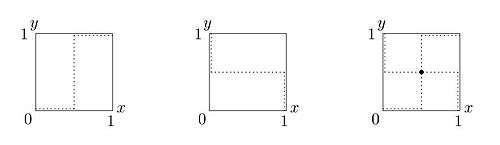Figure 7. Reaction correspondences for players in the matching pennies game. The leftmost mapping is for the coordinating player, the middle shows the mapping for the discoordinating player. The sole Nash equilibrium is shown in the right hand graph.

## Best response dynamics

In evolutionary game theory, best response dynamics represents a class of strategy updating rules, where players strategies in the next round are determined by their best responses to some subset of the population. Some examples include:

• In a large population model, players choose their next action probabilistically based on which strategies are best responses to the population as a whole.
• In a spatial model, players choose (in the next round) the action that is the best response to all of their neighbors (Ellison 1993).

Importantly, in these models players only choose the best response on the next round that would give them the highest payoff on the next round. Players do not consider the effect that choosing a strategy on the next round would have on future play in the game. This constraint results in the dynamical rule often being called myopic best response.

In the theory of potential games, best response dynamics refers to a way of finding a Nash equilibrium by computing the best response for every player:

Theorem: In any finite potential game, best response dynamics always converge to a Nash equilibrium. (Nisan et al. 2007, Section 19.3.2)

## Smoothed best response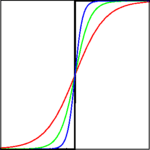Figure 8. A BR correspondence (black) and smoothed BR functions (colors)

Instead of best response correspondences, some models use smoothed best response functions. These functions are similar to the best response correspondence, except that the function does not "jump" from one pure strategy to another. The difference is illustrated in Figure 8, where black represents the best response correspondence and the other colors each represent different smoothed best response functions. In standard best response correspondences, even the slightest benefit to one action will result in the individual playing that action with probability 1. In smoothed best response as the difference between two actions decreases the individual's play approaches 50:50.

There are many functions that represent smoothed best response functions. The functions illustrated here are several variations on the following function:whererepresents the expected payoff of action, andis a parameter that determines the degree to which the function deviates from the true best response (a largerimplies that the player is more likely to make 'mistakes').

There are several advantages to using smoothed best response, both theoretical and empirical. First, it is consistent with psychological experiments; when individuals are roughly indifferent between two actions they appear to choose more or less at random. Second, the play of individuals is uniquely determined in all cases, since it is a correspondence that is also a function. Finally, using smoothed best response with some learning rules (as in Fictitious play) can result in players learning to play mixed strategy Nash equilibria (Fudenberg & Levine 1998).